# Math in Focus Grade 6 Chapter 2 Review Test Answer Key

Go through the Math in Focus Grade 6 Workbook Answer Key Chapter 2 Review Test to finish your assignments.

## Math in Focus Grade 6 Course 1 A Chapter 2 Review Test Answer Key

Concepts and Skills

Write the opposite of each number.

Question 1.
-47
The opposite number of -47 is 47.

Question 2.
56
The opposite number of 56 is -56.

Question 3.
-78
The opposite number of -78 is 78.

Draw a horizontal number line to represent each set of numbers.

Question 4.
-41, -37, -34, -30, -28, -25, -22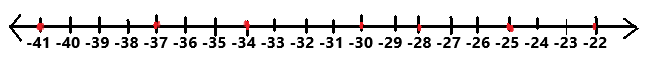The given numbers -41, -37, -34, -30, -28, -25, -22 are represented in the above horizontal number line. On a horizontal number line, the numbers become greater as you move to the right, and less as you move to the left.

Question 5.
-133, -129, -126, -122, -119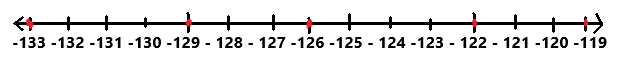The given numbers -133, -129, -126, -122, -119 are represented in the above horizontal number line. On a horizontal number line, the numbers become greater as you move to the right, and less as you move to the left.

Draw a vertical number line to represent each set of numbers.

Question 6.
-8, -6, -2, 1, 3, 4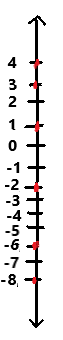The given numbers -8, -6, -2, 1, 3, 4 are represented in the above vertical number line. On a vertical number line, the numbers become greater as you move up, and lesser as you move down.

Question 7.
Odd numbers greater than -40 but less than -28.
Odd numbers greater than -40 but less than -28 are -39, -37, -35, -33, -31, -29.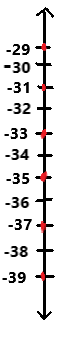The odd numbers greater than -40 but less than -28 are -39, -37, -35, -33, -31, -29 are represented in the above vertical number line. On a vertical number line, the numbers become greater as you move up, and lesser as you move down.

Write a positive or negative number to represent each situation.

Question 8.
A deposit of $94 Answer:$94
Explanation:
The given situation is a deposit of $94. The situation is represented with positive number$94.

Question 9.
181°F below zero.
-181°F
Explanation:
The given situation is 181°F below zero. Below represents negative number and above represents positive number. So the situation is represented with negative number -181°F.

Question 10.
The plane’s altitude is 23,920 feet
23,920 feet
Explanation:
The given situation is the plane’s altitude is 23,920 feet. The situation is represented with positive number 23,920 feet.

Question 11.
The elevation of a sunken ship that is 11 meters beneath the ocean’s surface
-11 meters
Explanation:
The given situation is the elevation of a sunken ship that is 11 meters beneath the ocean’s surface. Here the word beneath represents negative sign. So the situation is represented with negative number -11 meters.

Question 12.
A gain of 35 yards
35 yards
Explanation:
The given situation is a gain of 35 yards. The word gain represents a positive sign. The situation is represented with positive number 35 yards.

Copy and complete each Inequality using > or <.

Question 13.
-14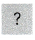-18
-14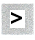-18
Explanation:
The given numbers are -14 and -18. Here we have to compare both the numbers. By comparing the above given numbers the number -14 is greater than -18.

Question 14.
17-11
17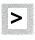-11
Explanation:
The given numbers are 17 and -11. Here we have to compare both the numbers. By comparing the above given numbers the number 17 is greater than -11.

Question 15.
-3423
-34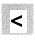23
Explanation:
The given numbers are -34 and 23. Here we have to compare both the numbers. By comparing the above given numbers the number -34 is less than 23.

Question 16.
-157-145
-157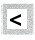-145
Explanation:
The given numbers are -157 and -145. Here we have to compare both the numbers. By comparing the above given numbers the number -157 is less than -145.

Order the numbers in each set.

Question 17.
Order the numbers from greatest to least:
15, -14, 7, 2, -5, -6, -9
The number from greatest to least: 15, 7, 2, -5, -6, -9, -14.

Question 18.
Order the numbers from least to greatest: 112, -140, -50, 51, -122, 175, -182
The numbers from least to greatest: -182, -140, -122, -50, 51, 112, 175.

Write an Inequality for each of the following statements using > or <.

Question 19.
-112°C is warmer than -143°C.
-112°C > -143°C
Explanation:
The given situation is -112°C is warmer than -143°C. Here we have to compare both the temperatures. After comparing the temperatures -112°C is greater than -143°C.

Question 20.
The lowest recorded temperature yesterday was -4°C, which is colder than today’s lowest recorded temperature of 4°C.
-4°C < 4°C
Explanation:
After comparing the above given temperatures and situation -4°C is less than 4°C.

Write the absolute value of each number.

Question 21.
|79|
The absolute value of the number |79| is 79.

Question 22.
|-88|
The absolute value of the number |-88| is 88.

Question 23.
|-102|
The absolute value of the number |-102| is 102.

Copy and complete each inequality using > or <.

Question 24.
|-65||-57|
|-65|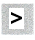|-57|
Explanation:
The absolute value of the number |-65| is 65.
The absolute value of the number |-57| is 57.
So, the number |-65| is greater than |-57|.

Question 25.
|111||-124|
|111|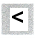|-124|
Explanation:
The absolute value of the number |111| is 111.
The absolute value of the number |-124| is 124.
So, the number |111| is less than |-124|.

Question 26.
|-153||135|
|-153|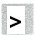|135|
Explanation:
The absolute value of the number |-153| is 153.
The absolute value of the number |135| is 135.
So, the number |-153| is greater than |135|.

Question 27.
|-209||-278|
|-209|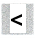|-278|
Explanation:
The absolute value of the number |-209| is 209.
The absolute value of the number |-278| is 278.
So, the number |-209| is less than |-278|.

Problem Solving

Question 28.
The Afar Depression is a land formation in Africa. At one location in the Afar Depression, the elevation is -75 meters. At another location, the elevation is -125 meters. Write an inequality to compare the elevations. Which elevation is farther from sea level?
-75 meters > -125 meters
The elevation -125 meters is farther from sea level.
Explanation:
At one location in the Afar Depression, the elevation is -75 meters.
At another location, the elevation is -125 meters.
After comparing the above elevations -75 meters is greater than -125 meters.

Question 29.
The table shows temperature readings taken at the same location at three different times.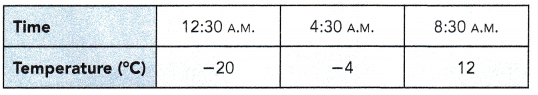a) At what time was the location the coldest?
At 12:30 A.M. the location is coldest.

b) Between 12:30 A.M. and 8:30 A.M., the temperature was always rising. Between what two times shown in the table did the temperature reach 0°C?
Between 4:30 A.M. and 8:30 A.M. the temperature reaches to 0°C as we can observe in the above table.

Question 30.
Clarence owes his brother Joe $240, and his best friend Tristan$166. His sister Chloe owes Clarence $275, and his friend Luke owes Clarence$150.
a) Clarence writes the number -240 to represent the amount he owes his brother Joe. What numbers should Clarence use to represent the other amounts given above?
Clarence owes the most money($240 +$166 = $406). c) How much does Clarence owe in total? Answer: Clarence owes$406 in total.
The total amount that people owes to Clarence is $275 +$150 = $475 The total amount that Clarence owes to others is$240 + $166 =$406